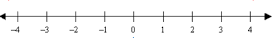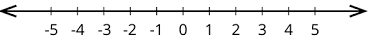# AP 6th Maths Mock Test 2021 For Chapter – 4 : ” Integers ” Online Practice Test

Choose Your Language: বাংলা, English, ગુજરાતી, हिन्दी, ಕನ್ನಡ, മലയാളം, मराठी, ਪੰਜਾਬੀ, தமிழ், తెలుగు,
1. Integers are combination of
2. Zero is
3. The integers are denoted by letter
4. Who first used a special sign for negative numbers ?
5. Zehlen means
6. Zehlen is a .......... word
7. Which of the following is not a Positive number ?
8. Which of the following is not a Negative number ?
9. Which number is neither positive nor negative ?
10. Represent the following with integers : A gain of ₹304
11. Represent the following with integers : Temperature is below 90C
12. Represent the following with integers : Temperature is above 80C
13. Represent the following with integers : Temperature is below 40C
14. Represent the following with integers : profit ₹234
15. Which of the following is a situation to represent positive integer ?
16. The following represents a number line of17. The smallest positive integer is
18. The greatest positive integer is
19. The greatest negative integer is
20. The smallest negative integer is
21. Positive integers are called
22. The temperature lie between 00 C and - 30 C23. The temperature lie between 00 C and 30 C24. Represent opposite integer of - 30  on number line
25. Represent opposite integer of 5  on number line
26. Find the correct statement on - 5 , - 6
27. -6 lies between
28. How many integers are there between 7 and - 7 ?
29. How many integers are there between 7 and 8 ?
30. -  34 lies between
31. Find the correct statement about 8 and 9
32.Which is the nearest positive integer to -1 ?
33. How many negative numbers you will find on the left side of zero ?34. How many negative numbers you will find on the right side of zero ?35. How many integers are there between - 1 and 5 ?36. How man negative integers are there between - 2 and 10 ?
37. How man positive integers are there between - 2 and 5 except 0 ?
38. Which of the following are less than -8 ?
39. Arrange the following integers in ascending order : 0 , - 5 , 8 , - 3 , 1
40. Arrange the following integers in ascending order : 0 , - 9 , 2 , - 8 , 1
41. Arrange the following integers in descending order : 2 , - 3 , 4 , - 5 , 6
42. Arrange the following integers in descending order : - 9 , - 8 , - 7 , - 6
43. The integer either side of 3 are
44. The integer either side of 0 are
45. -1 ........... 0
46. -100 ........... - 10000
47. -100 ........... 99
48. Find false statement
49. Find true statement
50. A : 0 is neither negative nor positive
B : Integers are countable
51. A : 0 is on the right of - 3 on number line
B : 56 > - 10
52. A : The integers on either side of - 5 are - 7 and - 4
B : Integers are combination of natural and whole numbers
53. The temperature recorded as follows : Shimla = - 40 C , Kufri = - 60 , Ooty = - 80 C , Gao = - 100 C then which is the colder place ?
54. Any two distinct numbers that give zero when added to each other are
55. Additive inverse of - 9 is
56. Additive inverse of 10 is
57. In additive inverse the sum of two numbers gives
58. Find the value of (- 9) - 6
59. Find the value of (- 5) + 45
60. Find the value of 7 - (- 9)
61. The sum of two positive integers are always
62. The sum of two negative integers are always
63. The sum of one positive integer and negative integer are always
64. The sum of - 9 + (- 2) is reveals
65. The sum of 10 + 12 produced
66. The sum of (- 20) , (- 82) , (- 28) and (- 14) is
67. The sum of 25 , (- 21) , (- 20) , 17 , (- 1) is
68. Simplify (- 6) + (- 10) + 5 + 17
69. Simplify 30 + (- 30) + (- 60) + (- 18)
70. Simplify 30 + (- 90) + 0
71. Subtract - 5 from 6 is
72. Subtract - 7 from - 9 is
73. Subtract 9 from 9 is
74. Sum of any two integers is
75. Integers are .................... under addition
76. - 19 + ........... = 0 the blank is occupied by
77. ........ + (12 - 16) = 0
78. ........ + (18 - 14) = 0
79. 90 + (- 90) =
80. (a + b) + c = a + ( b + c) it is
81. 9 - 4  ...... 2 - (- 3)
82. 2 - 3 ........ 3 - 2
83. 10 - 8 =
84. .................... greater than negative integers
85. All whole numbers are
86. Write all integers lie between - 8 and - 5
87. Write the following in ascending order : - 90 , - 10 , - 100
88. Write the following in descending order : - 70 , - 80 , - 90
89. Find the greatest among the following
90. Find the smallest among the following
91. Team A : - 90 , 56 , 87 , - 100 & Team B : 34 , - 100 , 98 , - 45 then who scored highest ?
92. The cost of 1 table is ₹456 but he sold it for ₹450 then how much loss he get ?
93. The profit is indicated by
94. The loss is indicated by
95. Which of the following is not an integer ?
96. Integers does not have
97. Which of the following has more numbers ?
98. Z = { ......... - 1 , 0 , 1 .............} it is a set of
99. The same meaning of number is
100. Which of the following is matched set for Z =